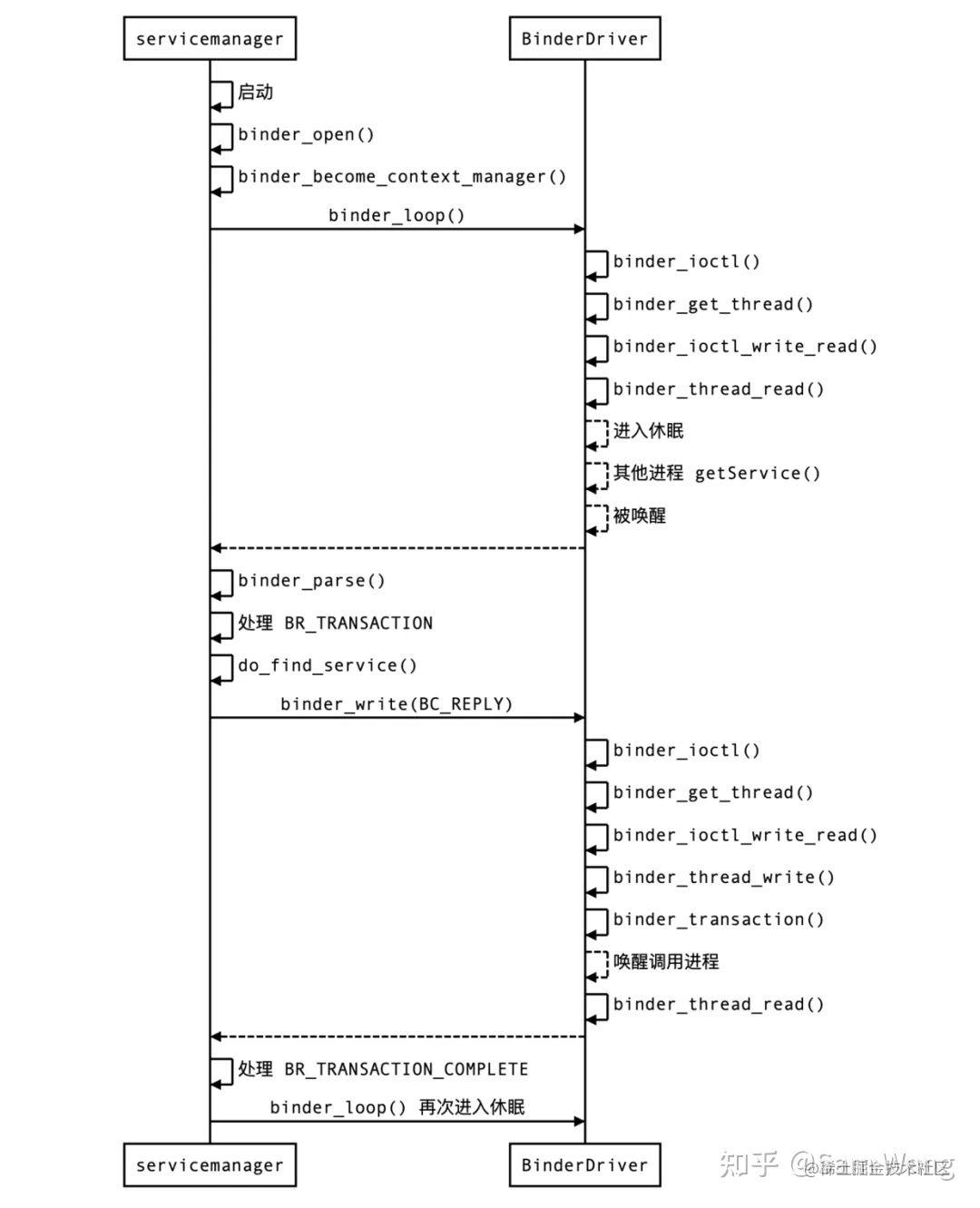# 掌握 binder 机制？驱动核心源码详解

❝本文设计源码及逻辑较多，已尽量画图辅助，完全理解可能要耗一定精力，建议收藏。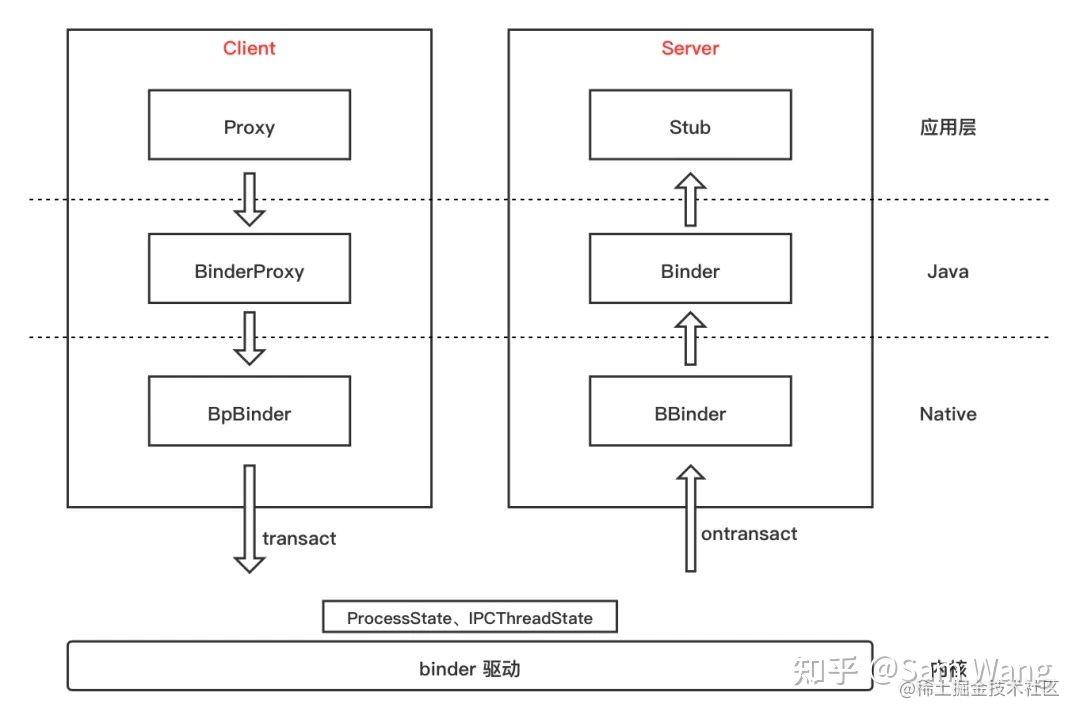binder 驱动的整体分层如上图，下面先来宏观的了解下 getService() 在整个 Android 系统中的调用栈，ServiceManager 本身的获取：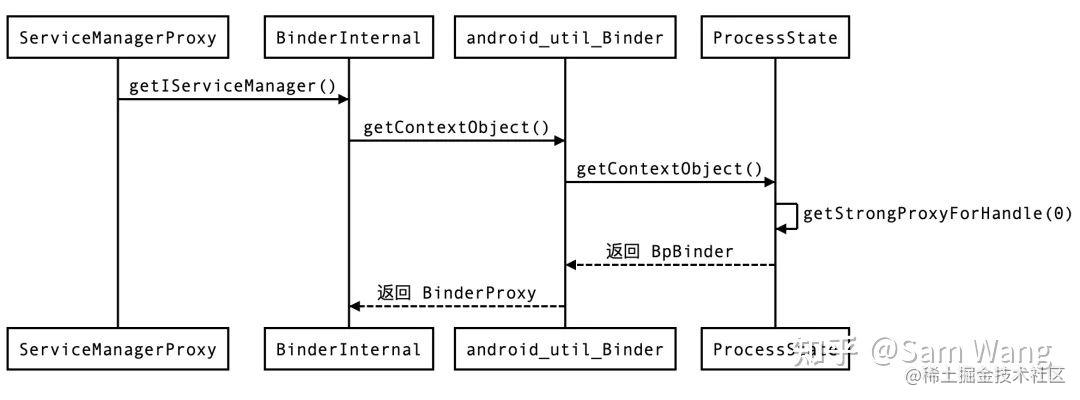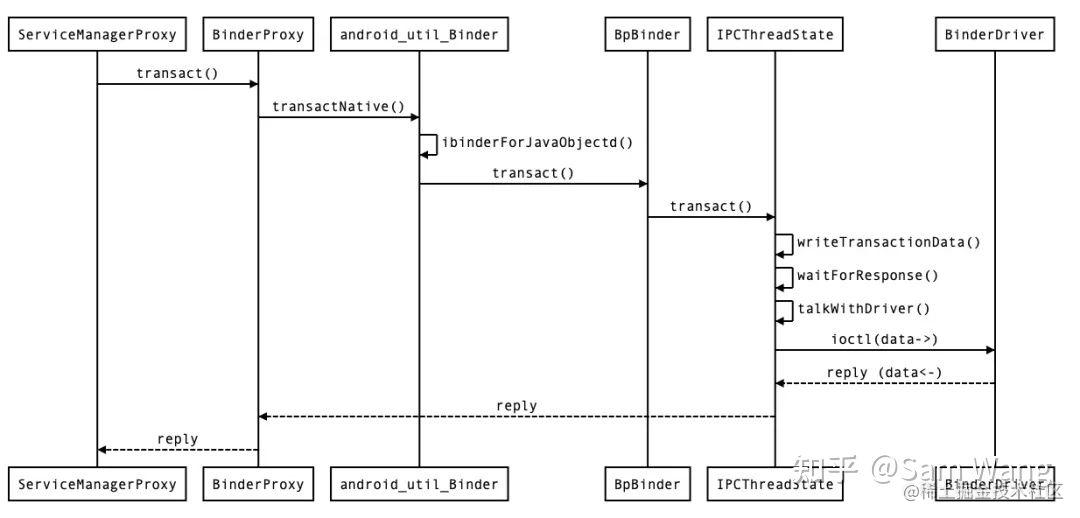「本文将主要分析此过程中 binder 驱动具体承担了哪些工作」，也就是上图中 IPCThreadState 与 binder 驱动的 ioctl 调用。

binder 驱动中做的工作可以总结为以下几步：

1. 准备数据，根据命令分发给具体的方法去处理
2. 找到目标进程的相关信息
3. 将数据一次拷贝到目标进程所映射的物理内存块
4. 记录待处理的任务，唤醒目标线程
5. 调用线程进入休眠
6. 目标进程直接拿到数据进行处理，处理完后唤醒调用线程
7. 调用线程返回处理结果

1. binder_ioctl()
5. binder_transaction()

## 1.binder_ioctl()

``````ioctl(mProcess->mDriverFD, BINDER_WRITE_READ, &bwr)

``````static long binder_ioctl(struct file *filp, unsigned int cmd, unsigned long arg){
int ret;
//拿到调用进程在 binder_open() 中记录的 binder_proc
struct binder_proc *proc = filp->private_data;
binder_lock(__func__);
switch (cmd) {
//处理 binder 数据读写,binder IPC 通信的核心逻辑
if (ret)
goto err;
break;
...
}

1. 创建及初始化每个进程独有一份的、用来存放 binder 相关数据的 binder_proc 结构体
2. 「将 binder_proc 记录起来，方便后续使用」

``````static int binder_open(struct inode *nodp, struct file *filp){
...
filp->private_data = proc;
...
}

``````static struct binder_thread *binder_get_thread(
struct binder_proc *proc){
struct rb_node *parent = NULL;
//从 proc 中获取红黑树根节点
//查找 pid 等于当前线程 id 的thread，该红黑树以 pid 大小为序存放
while (*p) {
parent = *p;
//current->pid 是当前调用线程的 id
p = &(*p)->rb_left;
p = &(*p)->rb_right;
else
break;
}

if (*p == NULL) {//如果没有找到，则新创建一个
return NULL;
}
}

``````static int binder_ioctl_write_read(struct file *filp,
unsigned int cmd, unsigned long arg,
int ret = 0;
struct binder_proc *proc = filp->private_data;
unsigned int size = _IOC_SIZE(cmd);
//用户传下来的数据赋值给 ubuf
void __user *ubuf = (void __user *)arg;
//把用户空间数据 ubuf 拷贝到 bwr
if (copy_from_user(&bwr, ubuf, sizeof(bwr))) {
ret = -EFAULT;
goto out;
}
暂时忽略处理数据逻辑...
//将读写后的数据写回给用户空间
if (copy_to_user(ubuf, &bwr, sizeof(bwr))) {
ret = -EFAULT;
goto out;
}
out:
return ret;
}

``````    if (bwr.write_size > 0) {//写数据
bwr.write_buffer, bwr.write_size,
&bwr.write_consumed);
trace_binder_write_done(ret);
if (ret < 0) { //写失败
if (copy_to_user(ubuf, &bwr, sizeof(bwr)))
ret = -EFAULT;
goto out;
}
}
filp->f_flags & O_NONBLOCK);
if (!list_empty(&proc->todo))
//唤醒等待状态的线程
wake_up_interruptible(&proc->wait);
if (ret < 0) { //读失败
if (copy_to_user(ubuf, &bwr, sizeof(bwr)))
ret = -EFAULT;
goto out;
}
}

``````status_t IPCThreadState::writeTransactionData(int32_t cmd, uint32_t binderFlags,
int32_t handle, uint32_t code, const Parcel& data, status_t* statusBuffer){
binder_transaction_data tr; //到驱动内部后会取出此结构体进行处理
tr.target.ptr = 0;
tr.target.handle = handle; //目标 server 的 binder 的句柄
//请求码，getService() 服务对应的是 GET_SERVICE_TRANSACTION
tr.code = code;
tr.flags = binderFlags;
tr.sender_pid = 0;
tr.sender_euid = 0;
const status_t err = data.errorCheck(); //验证数据合理性
if (err == NO_ERROR) {
tr.data_size = data.ipcDataSize(); //传输数据大小
tr.data.ptr.buffer = data.ipcData(); //传输数据
tr.offsets_size = data.ipcObjectsCount()*sizeof(binder_size_t);
tr.data.ptr.offsets = data.ipcObjects();
} else {...}
mOut.writeInt32(cmd); // transact 传入的 cmd 是 BC_TRANSACTION
mOut.write(&tr, sizeof(tr)); //打包成 binder_transaction_data
return NO_ERROR;
}

``````    bwr.write_buffer = (uintptr_t)mOut.data();

``````static int binder_thread_write(struct binder_proc *proc,
binder_uintptr_t binder_buffer, size_t size,
binder_size_t *consumed){
uint32_t cmd;
void __user *buffer = (void __user *)(uintptr_t)binder_buffer;
void __user *ptr = buffer + *consumed; //数据起始地址
void __user *end = buffer + size; //数据结束地址
//可能有多个命令及对应数据要处理，所以要循环
while (ptr < end && thread->return_error == BR_OK) {
if (get_user(cmd, (uint32_t __user *)ptr)) // 读取一个 cmd
return -EFAULT;
//跳过 cmd 所占的空间，指向要处理的数据
ptr += sizeof(uint32_t);
switch (cmd) {
case BC_TRANSACTION:
//与 writeTransactionData 中准备的数据结构体对应
struct binder_transaction_data tr;
//拷贝到内核空间 tr 中
if (copy_from_user(&tr, ptr, sizeof(tr)))
return -EFAULT;
//跳过数据所占空间，指向下一个 cmd
ptr += sizeof(tr);
//处理数据
break;
}
处理其他 BC_XX 命令...
}
//被写入处理消耗的数据量，对应于用户空间的 bwr.write_consumed
*consumed = ptr - buffer;

binder_thread_write() 中从 bwr.write_buffer 中取出了 cmd 和 cmd 对应的数据，进一步交给 binder_transaction() 处理，需要注意的是，BC_TRANSACTION、BC_REPLY 这两个命令都是由 binder_transaction() 处理的。

## 5.binder_transaction

binder_transaction() 方法中代码较长，先总结它干了哪些事：对应开头列出的工作，此方法中做了非常关键的 2-4 步：

1. 找到目标进程的相关信息
2. 将数据一次拷贝到目标进程所映射的物理内存块
3. 记录待处理的任务，唤醒目标线程

``````static void binder_transaction(struct binder_proc *proc,
struct binder_transaction *t; //用于描述本次 server 端要进行的 transaction
struct binder_work *tcomplete; //用于描述当前调用线程未完成的 transaction
binder_size_t *offp, *off_end;
struct binder_proc *target_proc; //目标进程
struct binder_node *target_node = NULL; //目标 binder 节点
struct list_head *target_list; //目标 TODO 队列
}else{
//处理 BC_TRANSACTION
if (tr->target.handle) { //handle 不为 0
struct binder_ref *ref;
//根据 handle 找到目标 binder 实体节点的引用
ref = binder_get_ref(proc, tr->target.handle);
target_node = ref->node; //拿到目标 binder 节点
} else {
// handle 为 0 则代表目标 binder 是 service manager
// 对于本次调用来说目标就是 service manager
target_node = binder_context_mgr_node;
}
}
target_proc = target_node->proc; //拿到目标进程
if (!(tr->flags & TF_ONE_WAY) && thread->transaction_stack) {
struct binder_transaction *tmp;
while (tmp) {
if (tmp->from && tmp->from->proc == target_proc)
tmp = tmp->from_parent;
}
}
target_list = &target_thread->todo; //拿到目标 TODO 队列

binder_transaction、binder_work 等结构体在上一篇中有介绍，上面代码中也详细注释了它们的含义。比较关键的是 binder_get_ref() 方法，它是如何找到目标 binder 的呢？这里暂不延伸，下文再做分析。

``````    t = kzalloc(sizeof(*t), GFP_KERNEL); //创建用于描述本次 server 端要进行的 transaction
tcomplete = kzalloc(sizeof(*tcomplete), GFP_KERNEL); //创建用于描述当前调用线程未完成的 transaction
if (!reply && !(tr->flags & TF_ONE_WAY)) //将信息记录到 t 中：
else
t->from = NULL;
t->to_proc = target_proc; //记录目标进程
t->code = tr->code; //记录请求码，getService() 对应的是 GET_SERVICE_TRANSACTION
t->flags = tr->flags;
//实际申请目标进程所映射的物理内存，准备接收要传输的数据
t->buffer = binder_alloc_buf(target_proc, tr->data_size,
tr->offsets_size, !reply && (t->flags & TF_ONE_WAY));
//申请到 t->buffer 后，从用户空间将数据拷贝进来，这里就是一次拷贝数据的地方！！
if (copy_from_user(t->buffer->data, (const void __user *)(uintptr_t)
tr->data.ptr.buffer, tr->data_size)) {
goto err_copy_data_failed;
}

`````` if (reply) { //如果是处理 BC_REPLY，pop 出来栈顶记录的 transaction(实际上是删除链表头元素)
} else if (!(t->flags & TF_ONE_WAY)) {
//如果不是 oneway，将 server 端要处理的 transaction 记录到当前调用线程
} else {
...暂不关注 oneway 的情况
}
t->work.type = BINDER_WORK_TRANSACTION;
tcomplete->type = BINDER_WORK_TRANSACTION_COMPLETE; //设置调用线程待处理的任务类型
if (target_wait)
wake_up_interruptible(target_wait); //唤醒目标线程

1. 准备数据，根据命令分发给具体的方法去处理
2. 找到目标进程的相关信息
3. 将数据一次拷贝到目标进程所映射的物理内存块
4. 记录待处理的任务，唤醒目标线程

1. 调用线程进入休眠
2. 目标进程直接拿到数据进行处理，处理完后唤醒调用线程
3. 调用线程返回处理结果

``````static int binder_thread_read(struct binder_proc *proc,
binder_uintptr_t binder_buffer, size_t size,
binder_size_t *consumed, int non_block){
void __user *buffer = (void __user *)(uintptr_t)binder_buffer; //bwr.read_buffer
void __user *ptr = buffer + *consumed; //数据起始地址
void __user *end = buffer + size; //数据结束地址
if (*consumed == 0) {
if (put_user(BR_NOOP, (uint32_t __user *)ptr))
return -EFAULT;
ptr += sizeof(uint32_t);
}
//是否要准备睡眠当前线程
wait_for_proc_work = thread->transaction_stack == NULL &&
if (wait_for_proc_work) {
if (non_block) { //non_block 为 false
ret = -EAGAIN;
} else
ret = wait_event_freezable_exclusive(proc->wait,
} else {
if (non_block) { //non_block 为 false
ret = -EAGAIN;
} else
}

consumed 即用户空间的 bwr.read_consumed，这里是 0 ，所以将一个 BR_NOOP 加到了 ptr 中。

``````static int binder_has_thread_work(struct binder_thread *thread){
}

``````struct binder_work *w;
switch (w->type) {
case BINDER_WORK_TRANSACTION_COMPLETE: {
cmd = BR_TRANSACTION_COMPLETE;
if (put_user(cmd, (uint32_t __user *)ptr))
return -EFAULT;
ptr += sizeof(uint32_t);
list_del(&w->entry); //删除 binder_work 在 thread->todo 中的引用
kfree(w);
}
case BINDER_WORK_NODE{...}
...

mIn 中有 BR_NOOP 和 BR_TRANSACTION_COMPLETE 两个命令，首先处理 BR_NOOP 命令，此命令什么也没做，由于 talkWithDriver() 处于 while 循环中，会再一次进入 talkWithDriver()，但因为此时 mIn 中还有数据没读完，不会调用 binder_ioctl()。

## 最后

1. 目标进程直接拿到数据进行处理，处理完后唤醒调用线程
2. 调用线程返回处理结果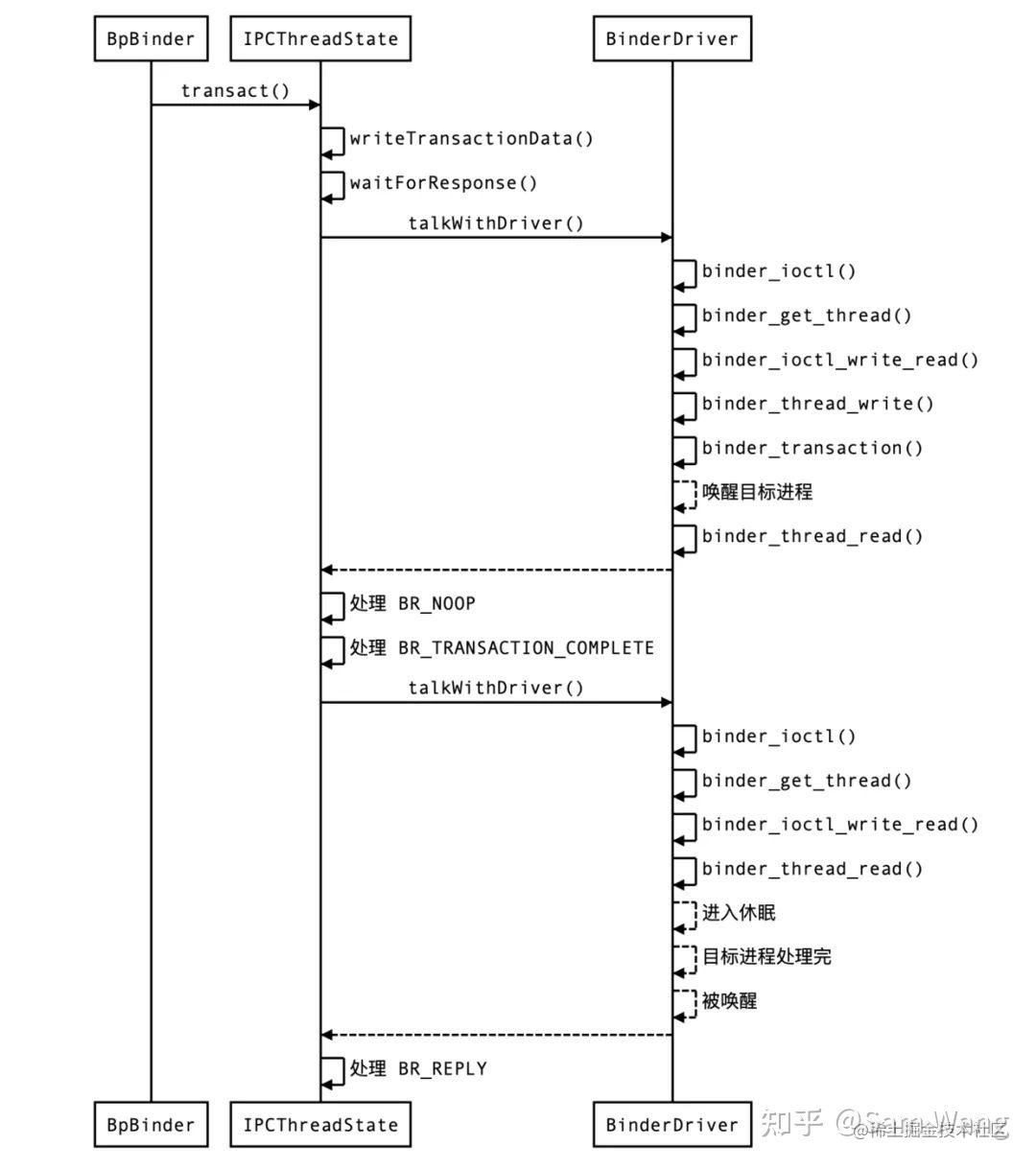Service Manager 端逻辑：